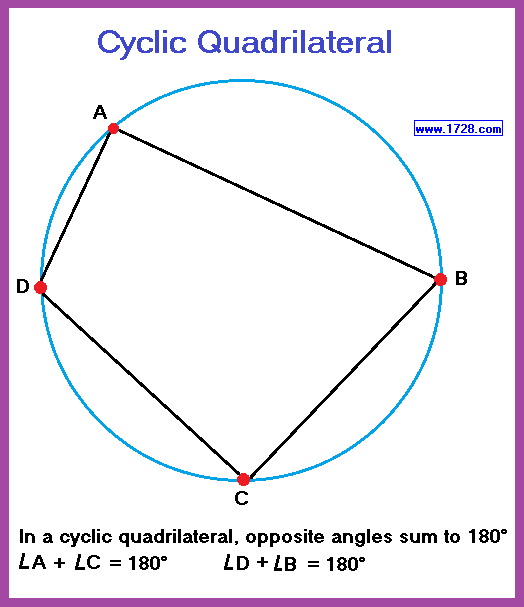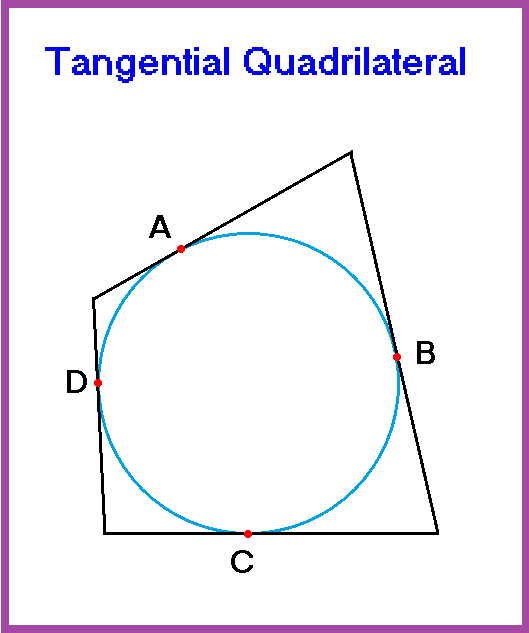Q u a d r i l a t e r a l s Scroll to the bottom for a listing ofproperties for all types of quadrilaterals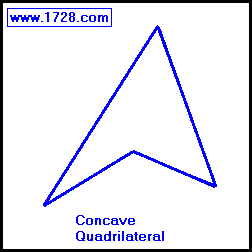A quadrilateral is a geometric figure having four sides and four angles which always total 360°.

We will discuss all types of quadrilaterals except the concave quadrilateral. (See diagram).

This type of quadrilateral has one angle greater than 180°. (Angles greater than 180° are called concave angles). These quadrilaterals are not discussed much in a typical geometry course and are not among the quadrilaterals with which you are familiar.

Generally, all a quadrilateral needs to be classified as such is four sides. However, there are six specific quadrilaterals that are worth discussing in detail.

Trapezoids

First, it is important to state the difference in definitions between British and American usage.
The British use the term trapezoid to refer to a quadrilateral with no parallel sides and a trapezium is a quadrilateral with two parallel sides.

The American usage is the exact opposite of the British usage: trapezoid - two parallel sides       trapezium - no parallel sides.

The only requirement for a trapezoid (American definition) is that two sides are parallel.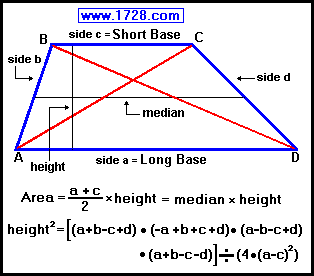Side a and side c are the parallel sides and are called bases.

The non-parallel sides (side b and side d) are called legs.

Lines AC and BD are the diagonals.

The median is perpendicular to the height and bisects lines AB and CD.

∠ A plus ∠ B = 180°     ∠ C plus ∠ D = 180°

Trapezoid Area = ½ • (sum of the parallel sides) • height

height² = [(a +b -c +d) • (-a +b +c +d) • (a -b -c +d) • (a +b -c -d)] ÷ (4 • (a -c)²)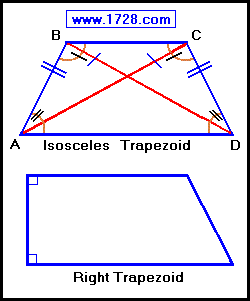Two special cases of trapezoid are worth mentioning.

The legs and diagonals of an isosceles trapezoid are equal.
AB = CD and AC = BD

Both pairs of base angles are equal
∠ A = ∠ D and ∠ B = ∠ C

The right trapezoid has two right angles.

Kites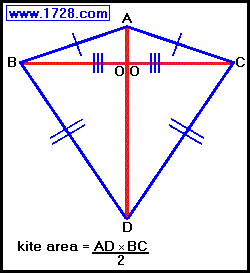∠ A and ∠ D are vertex angles

∠ B = ∠ C and are the non-vertex angles

Lines AD and BC are diagonals and always meet at right angles.

Diagonal AD is the axis of symmetry and bisects diagonal BC, bisects ∠ A and ∠ D, and bisects the kite into two congruent, triangles. (△ ABD and △ ACD)

Diagonal BC bisects the kite into two isosceles triangles. (△ ABC and △ BCD)

Side AB = side AC, side BD = side CD and Line OB = Line OC

Parallelograms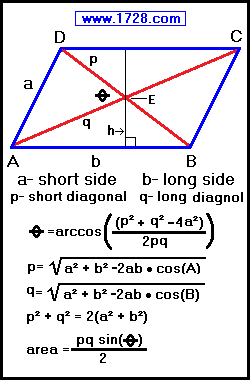• Both pairs of opposite sides are equal. (Side AD = BC     Side AB = DC)
• Both pairs of opposite angles are equal (∠ A = ∠ C     ∠ D = ∠ B)
• Diagonals bisect each other.   AE = EC     DE = EB
• Each diagonal forms two congruent triangles.
Diagonal AC forms △ ADC and △ ABC
Diagonal DB forms △ DCB and △ DAB
• Diagonals are not equal.
• Diagonals do not bisect the vertex angles.
• Area = side b • height   or   area = Line AB • height
• Angle A = arc sine (height ÷ side a)
• Angle A = arc cosine (side a² + side b² -p²) ÷ (2 • side a • side b)
• Angle B = (180° -Angle A)
• Side a = Square Root [ (p² + q² -2b²) / 2 ]
• Side b = Square Root [ (p² + q² -2a²) / 2 ]
• Height = side a • sine (A)
• Perimeter = 2 • (side a + side b)

Rhombuses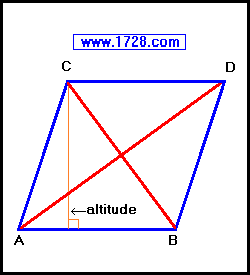• All four sides are equal (Side AB = BD = DC = CA)
• Diagonals meet at right angles
• Diagonals bisect each other
• Diagonals bisect the vertex angles   ∠ A   ∠ B   ∠ C   and   ∠ D
• Both pairs of opposite angles are equal
∠ A = ∠ D     ∠ C = ∠ B
• Adjacent angles are supplementary (they sum to 180°)
∠ A + ∠ B   =   ∠ B + ∠ D   =   ∠ D + ∠ C   =   ∠ C + ∠ A     = 180°
• Rhombus Altitude = side • sine (either angle)   =   (AD • CB) ÷ (2 • side)
• Rhombus Area = (AD × CB) ÷ 2   =   side • altitude   =   side² • sine (either angle)
• Long Diagonal AD = Side Length • Square Root [ 2 + 2 • cos(A) ]
• Short Diagonal BC = Side Length • Square Root [ 2 + 2 • cos(B) ]
• 4 • Side² = Long Diagonal² + Short Diagonal²

Rectangles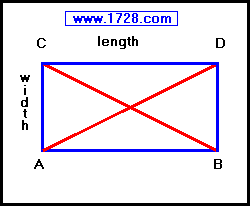• Opposite sides are parallel and equal

• All 4 angles are right angles

• Diagonals bisect each other and are equal

• Rectangle Area = length × width

• Perimeter = (2 × length) + (2 × width)

Squares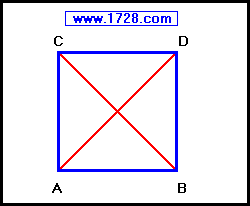• All 4 sides are equal

• All 4 angles are right angles

• Diagonals bisect each other at right angles and are equal

• Perimeter = 4 × side length

• Area = (side length)2